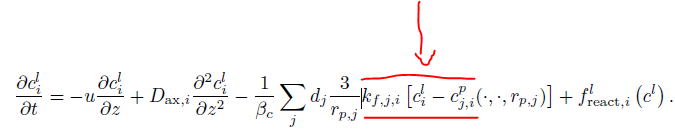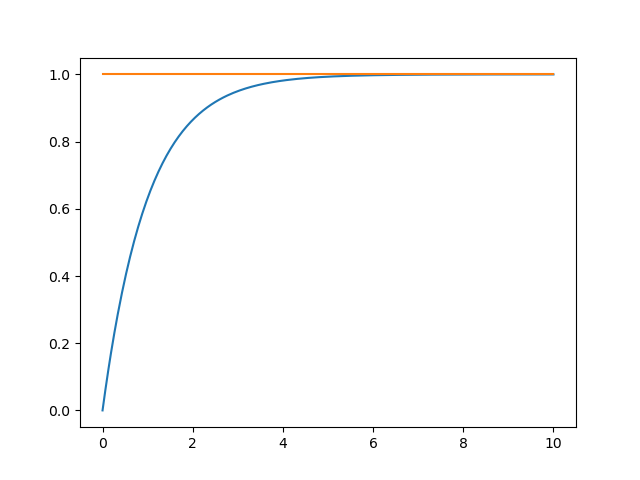# GRM and CSTR as Gas-Liquid Contactor/Reactor

Hi all!

I’d like to use CADET to model a gas-liquid reaction system. In general, mass transfer between these phases is given by k_{L}a(c*-c).

1. Is it possible to substitute the k_{L}a term for the red marked term in a GRM below?2. How does it look like for a CSTR?

Thanks a lot!
Jan

Hey Jan and welcome to the forum!The term c_{j,i}^p stands for the mobile phase concentration of the ith component in the jth particle type (see also here). Currently, the CSTR does not support particle pores because we initially assumed that all mobile phase is well mixed in a CSTR. However, we might change that in future. There is already an issue on Github.

But even if that were to be implemented, I’m not sure this would solve your problem. What you could try instead is to make use an isotherm model. These are usually meant to describe mass transfer from a liquid phase to a solid phase c_{j,i}^s but if the math is the same, it should still work!For example, the linear isotherm model has two mass transfer parameters LIN_KA and LIN_KD which quantify the transfer from liquid to solid and back.
However, I’m not sure how to proceed from there. What are you planning to do with the mass in the other phase? Unfortunately, we have no straight forward means of transporting that mass from one unit to another unit.

In the past, we have also already discussed counter-current methods before (see this issue). Being able to have independently moving phases (solid, liquid, or gas) would enable modelling many other processes like expanded bed adsorption, liquid-liquid chromatography, extraction processes, or gas-liquid systems as you are describing.
Having said that, we currently do not have the resources to implement that in a near future.

Since CADET is open source, feel free to submit ideas or, even better, pull requests should you consider implementing some of these features yourself.

Hi Jan,

Can you perhaps send a few more details or a paper with the system and equations you want to solve? Without further information it is hard to say if we can provide you with an easy solution to your problem.

Thanks,
Eric

Hi,
thank you for the quick responses.In more detail, I would like to set up a compartment reactor model, which exists of a plug flow reactor and a CSTR (PFR>CSTR). I have assumed that it could work with a set of GRM and CSTR in CADET (Inlet>GRM>CSTR>Outlet), but I don’t know how to implement the mass transfer (k_la term).

For a PFR, the following equation must be solved:

\frac{dc}{dt} = -u \frac{dc}{dt} + D_{ax} \frac{d^2c}{dx^2} + k_la(c^*-c) + \text{reaction part(if existing)}

For a CSTR,

\frac{dc}{dt} = \frac{1}{T} (c_{in} - c_{out}) + k_la(c^* - c) + \text{reaction part (if existing)}
\text{T: residence time}

must be solved.

The link to the other phase is given by the mass transfer k_la (c^*-c). c^* is the saturation concentration at the interface and is expressed by the partial pressure p_i in the gas phase and the henry coefficient. During the mass transport, the partial pressure within the gas phase can reduce. For the first, I would assume that the partial pressure p_i is constant and thus c^* too.

I hope this gives you a better description of my issues and once again, thank you very much for your support!

Best regards,
Jan

Hi Jan,

Instead of using the linear isotherm, we believe you can solve the system by introducing c^* as a separate (pseudo) component. By using chemical reactions, it is possible to model the mass transfer from one phase to another treating c^* as a modifier which affects the kinetics but is not consumed.

r_1 = k_la \cdot c^*
r_2 = - k_la \cdot c_{bulk}

For this purpose, we would set the stoichiometric coefficients to zero for c^* and manually manipulate the exponents matrix s.t. the component is still included in the mass balance of the reaction.

k_la = 1
reaction_parameters = Dict()
reaction_parameters.reaction_model = 'MASS_ACTION_LAW'
reaction_parameters.mal_kfwd_bulk = [k_la, k_la]
reaction_parameters.mal_kbwd_bulk = [0, 0]
reaction_parameters.mal_stoichiometry_bulk = [
1, -1,
0,  0
]
reaction_parameters.mal_exponents_bulk_fwd = [
0,  1,
1,  0
]


As can be seen in the figure below, c_{bulk} (blue) increases and c^* (orange) stays constant.To get started with chemical reactions, we have a tutorial here. If you need help implementing this idea for your system, just let me know, I’d be happy to assist.

Btw, if you use \$ surrounding your equations, then Discourse is able to render LaTeX syntax which helps readability.

1 Like

Hi,

Thank you! This is a great idea, I will try this approach and let you know if I need some help and if it works.